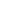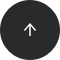Back to articles list- 5 minutes read

# How to Count XML Nodes in PostgreSQL 9.1

Recently I was given a task which involved counting, with a single SQL query, all tables in each model version of Vertabelo. Vertabelo internally stores each model version as an XML file (download a sample XML file). So my task was to count all XML nodes satisfying a certain XPATH expression. Vertabelo runs on PostgreSQL 9.1, so PostgreSQL 9.1 tools were all I could use.

To begin at the end, the final query looks like this:

```select id, coalesce(
array_length(
xpath(
'/DatabaseModel/Tables/Table',
xmlparse(content
convert_from(
'UTF-8'))),
1),
0) as table_count
from model;
```

Nice, isn’t it? And kind of complicated for such a simple task. Here is how I came up with the query.

A greatly simplified Vertabelo database schema looks like this.

In the table `model` there is a primary key `id` and a column `model_xml` which stores XML files with the model definition. The `model_xml` column type is OID.

There are two parts in the query: the part which handles XML and the part which handles OIDs.

### XML: how to use XPATH in PostgreSQL

The XML part of the query is this:

```select coalesce(array_length(xpath('/DatabaseModel/Tables/Table',
xmlparse(content XML_AS_TEXT_HERE)),
1), 0) as table_count
from model;
```

#### The xpath function

The essence of the query is the function xpath:

```xpath(xpath_exp, xml)
```

The function takes an XPATH expression and an XML value and evaluates the expression against the XML value. The XPATH expression is given as text. We’ll return to the XPATH expression in a minute. The xml value has to be given as an expression of type XML.

#### Parse XML with xmlparse

To convert a string expression into XML you can use the xmlparse function:

• `xmlparse(content STRING)` parse a chunk of XML
• `xmlparse(document STRING)` parse document XML

I chose the `xmlparse(content STRING) variant`, but in my case both variants would have worked.

#### The XPATH expression

Now to the XPATH expression. The Vertabelo XML file looks like this. The inessential parts have been left out.

```

...

purchase
...

...

```

The XPATH expression which selects all Table nodes is like this: `/DatabaseModel/Tables/Table`. I only wanted to count the nodes so in theory, I could use the XPATH expression `count(/DatabaseModel/Tables/Table)`. However, when you run this query against the PostgreSQL 9.1 database, you’ll get an empty array.

```select id, xpath('count(/DatabaseModel/Tables/Table)',
xmlparse(content
convert_from(
lo_open(model_xml, 262144), 10000000),
'UTF-8')))
as table_count
from model;
```

``` id | table_count
----+-------------
10 | {}
```

A little bit of Googling and you’ll find out why. Up till version 9.2 (we’re on 9.1, remember?) PostgreSQL has a bug: whenever the XPATH expression returns a scalar value, the database returns an empty array.

#### Use array_length

What can you do about it? Well, for non-scalar values, the database returns an array. So we can use PostgreSQL’s `array_length` function to count the nodes. The syntax is like this:

```array_length(array, dimension)
```

where dimension is the dimension of the array you want to measure. Our array is one-dimensional, so we set dimension to 1.

But... The array_length function returns null if the argument array is null. And we want to get 0. So we have to use `coalesce` (i.e., if exp is null then x else exp) expression to finish the XML part.

### OID: how I convert OID into string

The query would have been easier if the column `model_xml` were of type XML, or even text. Alas, the database architect has decided that the column would be of type OID. You know how much fun OIDs are in SQL queries. The only purpose of this horrendous expression is to convert OID into text:

```convert_from(
```

And it worked only because my query was a one-off. What’s going on here? Again, we read the query inside-out. The expression

```lo_open(model_xml, 262144)
```

opens the OID file in read-only mode. The magic number 262144 is a flag for the open mode, 40000 in hex, which means “open read-only”.

Then we have the function loread:

```loread(fd, len)
```

The function loread takes a file descriptor (i.e., the value which `lo_open` returns) and a buffer length and returns a BYTEA value with at most len data read from the file. I have taken the function from a comment on StackOverflow. The suggestion is to use the length 1000000 but the first time I tried it on my data it failed. So, I increased the limit to 10000000 (See? I told you it was a one-off!) and for my data it worked. I wish I knew how to convert it regardless of the size of my data.

#### From BYTEA to text

One more thing: the loread function returns a value of type BYTEA. But we want to have a text value for xmlparse.

The function to use is `convert_from`:

```convert_from(string bytea, src_encoding name)
```

The function takes a string as BYTEA value and the text encoding name end converts it into a text with database encoding.

And that’s it. The final query again:

```select id, coalesce(
array_length(
xpath(
'/DatabaseModel/Tables/Table',
xmlparse(content
convert_from(